# Saxon Calculus: Techniques of Antidifferentiation Chapter Exam

Exam Instructions:

Choose your answers to the questions and click 'Next' to see the next set of questions. You can skip questions if you would like and come back to them later with the yellow "Go To First Skipped Question" button. When you have completed the practice exam, a green submit button will appear. Click it to see your results. Good luck!

### Page 1

#### Question 1 1. Factor the fraction below.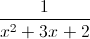#### Question 2 2. Evaluate the integral.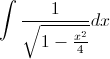#### Question 3 3. Evaluate the following integral.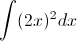#### Question 4 4. Evaluate the following integral.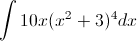#### Question 5 5. Evaluate and solve the following integral.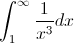### Page 2

#### Question 6 6. Evaluate the following integral.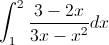#### Question 7 7. Integrate the following.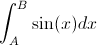#### Question 8 8. Evaluate the following.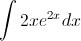#### Question 9 9. Use integration by parts to integrate the following: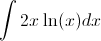#### Question 10 10. Evaluate the following integral.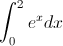### Page 3

#### Question 11 11. Simplify the following integral.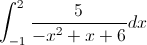#### Question 12 12. Use integration by part to integrate the following: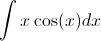#### Question 13 13. In the following equation, g(s) is the _____.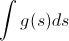#### Question 14 14. Integrate the following.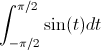#### Question 15 15. Factor the fraction below.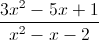### Page 4

#### Question 16 16. Integrate the following.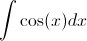#### Question 17 17. Evaluate and solve the following integral.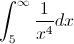#### Question 18 18. Evaluate the following integral.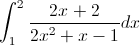#### Question 19 19. Which of the following expressions is equal to the integral below?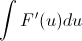#### Question 20 20. Evaluate the following integral.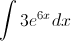### Page 5

#### Question 21 21. Evaluate the integral.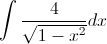#### Question 22 22. Evaluate the following integral.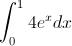#### Question 23 23. Evaluate the integral.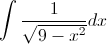#### Question 24 24. Evaluate the following.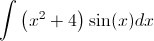#### Question 25 25. Evaluate and solve the following integral.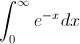### Page 6

#### Question 26 26. In the following equation, s is the _____.#### Question 27 27. Evaluate the following integral.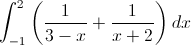#### Question 28 28. Evaluate the following integral.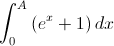#### Question 29 29. Evaluate and solve the following integral.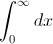#### Question 30 30. Factor the fraction below.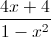#### Saxon Calculus: Techniques of Antidifferentiation Chapter Exam Instructions

Choose your answers to the questions and click 'Next' to see the next set of questions. You can skip questions if you would like and come back to them later with the yellow "Go To First Skipped Question" button. When you have completed the practice exam, a green submit button will appear. Click it to see your results. Good luck!

Support